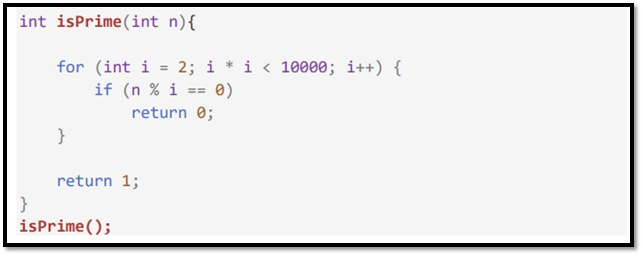### How to Calculate Time Complexity of an Algorithm + Solved Questions (With Notes)

Either you can download the handwritten notes in pdf (Link is given at the end of the page) or you can read them on this site itself.

#### Techniques to calculate Time Complexity

Once we are able to write the runtime in terms of the size of the input (n), we can find the time complexity.

For example,           T(n) = n2 → O (n2)

T(n) = log n → O (log n)

Some tricks to calculate the complexity

• Drop the constants: Anything you might think is O(3n) is O(n) [Better Representation]
• Drop the non-dominant terms: Anything you represent as O(n2+n) can be written as O(n2)
• Consider all variables which are provided as input: O (mn) and O (mnq) might exist for some cases.

In most cases, we try to represent the runtime in terms of the input which can be more than one in number. For example,

Painting a park of dimension m * n → O (mn)

#### Time Complexity – Competitive Practice Sheet

1. Fine the time complexity of the func1 function in the program show in program1.c as follows: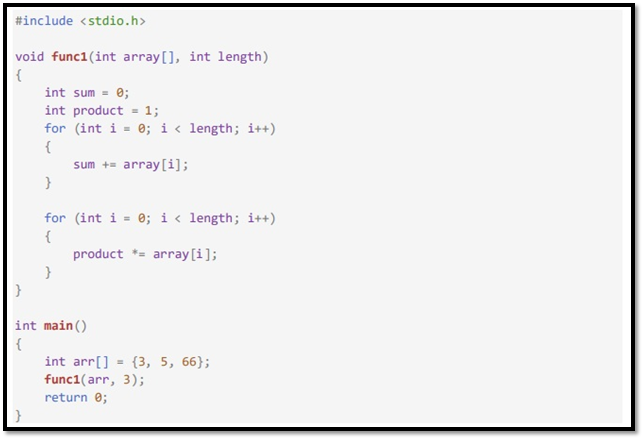2. Fine the time complexity of the func function in the program from program2.c as follows: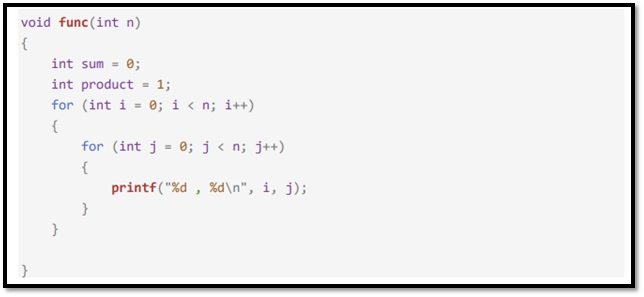3. Consider the recursive algorithm above, where the random(int n) spends one unit of time to return a
random integer which is evenly distributed within the range [0,n][0,n]. If the average processing time
is T(n), what is the value of T(6)?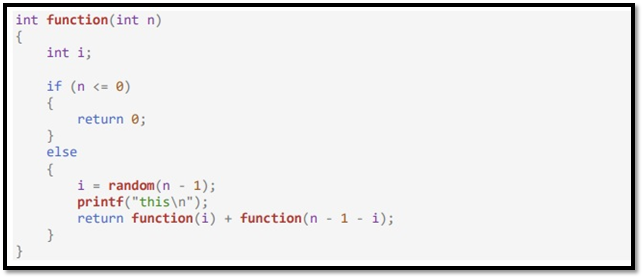4. Which of the following are equivalent to O(N)? Why?
a) O(N + P), where P < N/9
b) 0(9N-k)
c) O(N + 8log N)
d) O(N + M2)

5. The following simple code sums the values of all the nodes in a balanced binary search tree. What is its
runtime?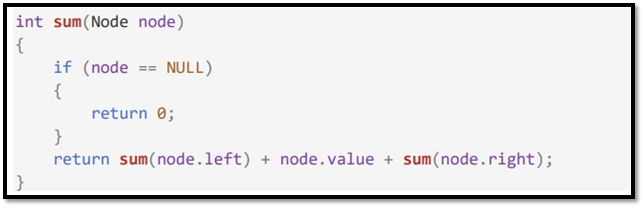6. Find the complexity of the following code which tests whether a give number is prime or not?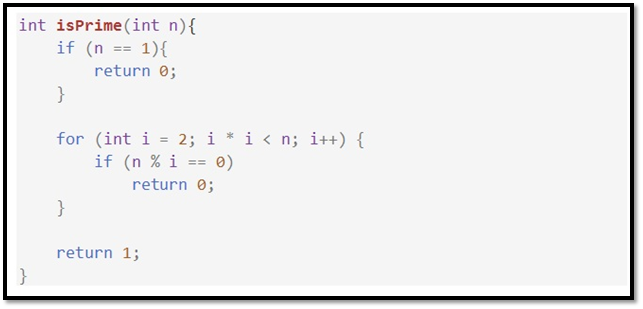7. What is the time complexity of the following snippet of code?